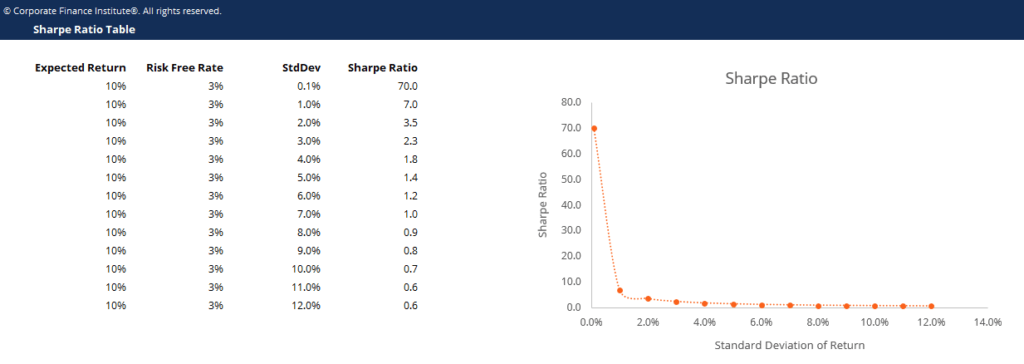# Sharpe Ratio Table Template

## Sharpe Ratio Table Template

This sharpe ratio table template demonstrates the calculation of sharpe ratio given the expected portfolio return, risk-free rate and standard deviation of the portfolio return.

Below is a screenshot of the sharpe ratio table template:### Sharpe Ratio Table Template

Sharpe Ratio (or Sharpe Index) is commonly used to calculate the performance of an investment by adjusting for its risk.

The higher the ratio, the greater the return of portfolio relative to the risk taken, and thus the better the investment. The ratio can be used to evaluate a single stock or investment, or an entire portfolio.

### Sharpe Ratio formula

Sharpe Ratio = (Rx – Rf) / StdDev Rx

Where:

• Rx = Expected portfolio return
• Rf = Risk free rate of return
• StdDev Rx = Standard deviation of portfolio return / volatility

It’s all about reducing volatility.  If an investment had an annual return of only 10%, but had zero volatility, it would have an infinite (or undefined) Sharpe Ratio.

Of course, it’s impossible to have zero volatility, even with a government bond (prices go up and down).  As volatility increases, the expected return has to go up significantly to compensate for that additional risk.

### More Free Templates

For more resources, check out our business templates library to download numerous free Excel modeling, PowerPoint presentation and Word document templates.

• Excel Modeling Templates
• PowerPoint Presentation Templates
• Transaction Document Templates

### Financial Analyst Certification

Become a certified Financial Modeling and Valuation Analyst (FMVA)® by completing CFI’s online financial modeling classes and training program!